### Modeling raw counts

A note on normalizing data

• Data normalization is done to determine is differences between data is due to reasons beyond biological and technical variance
• In previous notes, data was normalized to size factors in order to equalize the effects of high count and low count genes for analysis at the genome/transcriptome level
• When comparing two whole transcription profiles, want to make sure genes with particular levels of expression aren’t dominating the comparison
• For analysis at the gene level we do not need to normalize the same way, since the expression levels of other genes do not directly affect the comparison between samples for a single gene
• For gene level comparisons, we normalize by modeling feature counts from raw counts using the negative binomial distribution
• The negative binomial distribution is a dispersed variation of a Poisson distribution

Poisson distribution

• [Reminder] - The Poisson distribution is a discrete probability distribution modeling the probability of obtaining a certain “count” given an “average count” or “rate”
• The law of small numbers says that the sum of binary outcomes with a large $$N$$ and small $$p$$ can be modeled by a Poisson distribution
• RNAseq data fits this description since there are many outcomes (genes) and a small probability for each (% of individual gene out of all genes)
• For a single sample, the genes in the sample all follow a Poisson distribution
• So, in a pool of transcripts, every gene follows a Poisson distribution with a rate relative to how much of that specific transcript is available in the sample
• A result of this is that, for low-expression genes, the ratios of expression between samples are going to be much more variable than ratios of high expression genes
• However, this variability is due only to sampling variablity and does not take into account experimental or biological variability
• This can be seen when comparing mean expression to expression variance
• For a true poisson variable, variance is equal to the mean

x <- rpois(10000,lambda=100)
mean(x); sd(x)^2
##  100.0772
##  99.617
• However, with biological data, the variance usually exceeds the mean, indicating additional sources of variability
• When comparing ACROSS samples, genes in the samples do not follow a simple Poisson distribution

Negative binomial distribution

• The negative binomial distribution can be formalized as $$K_{ij} \sim \text{NB}(\mu_{ij}, \alpha_i)$$
• For RNA-seq data, more specifically formalized as $$K_{ij} \sim \text{NB}(s_{ij} q_{ij}, \alpha_i )$$
• $$K_{ij}$$ is the raw count of a particular gene $$i$$ for a particular sample $$j$$
• $$\text{NB}$$ is the negative binomial distribution
• $$s_{ij}$$ is a normalization factor (e.g. size factor) for gene $$i$$ in sample $$j$$
• $$q_{ij}$$ is the modeled count to be used for comparison for gene $$i$$ in sample $$j$$
• $$s_{ij}q_{ij}$$ is equivalent the the NB mean $$\mu_{ij}$$
• $$\alpha_i$$ is the dispersion factor
• The dispersion factor $$\alpha_i$$ determines how far the variation of the negative binomial distirbution exceeds that of the equivalent Poisson distribution

library(rafalib)
mypar(3,1)
n <- 10000
brks <- 0:400
hist(rpois(n,lambda=100),
main="Poisson / NB, disp=0",xlab="",breaks=brks,col="black")
hist(rnbinom(n,mu=100,size=1/.01), #size = 1/dispersion
main="NB, disp = 0.01",xlab="",breaks=brks,col="black")
hist(rnbinom(n,mu=100,size=1/.1),
main="NB, disp = 0.1",xlab="",breaks=brks,col="black")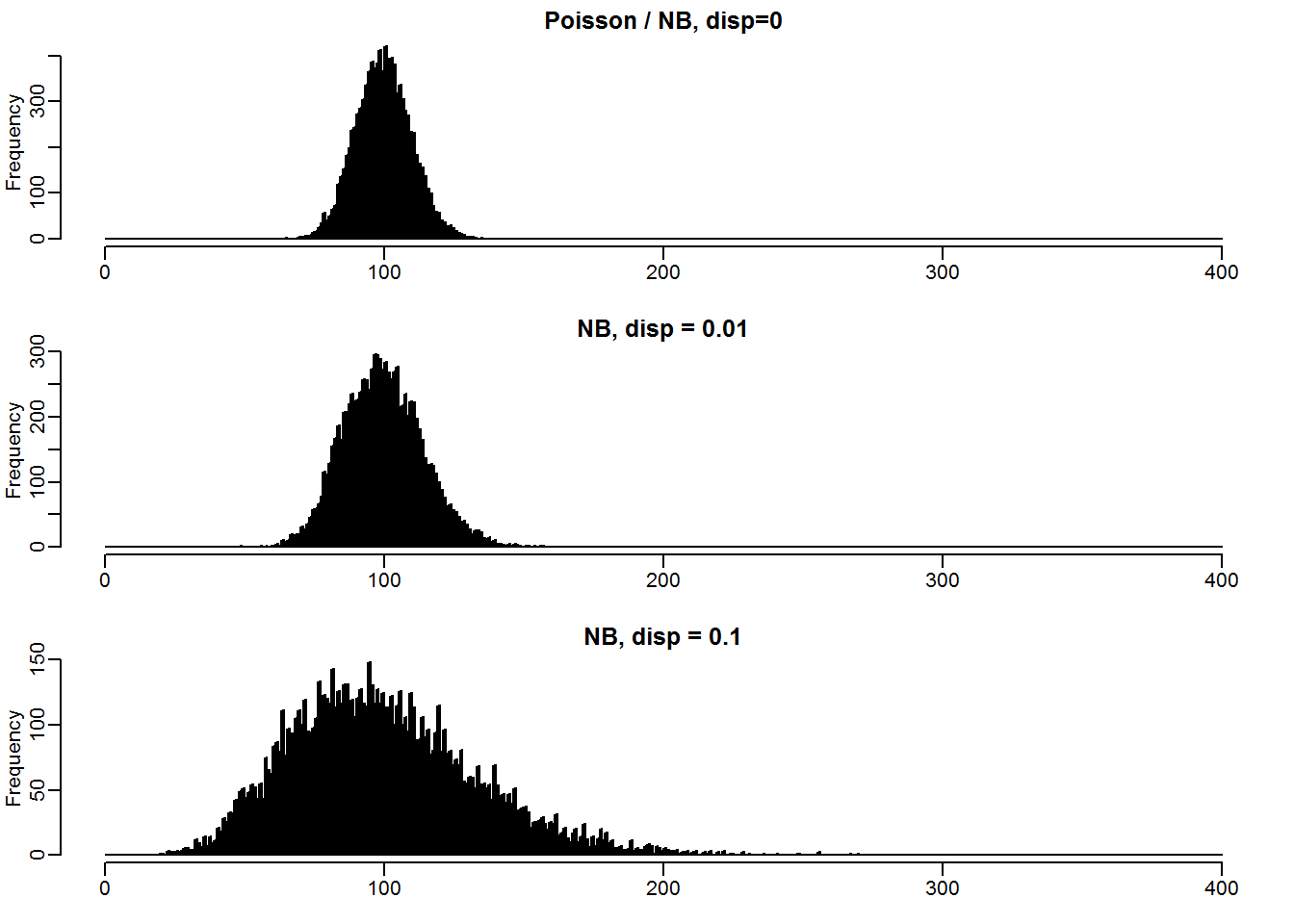• The variance of the negative binomial is equivalent to $$\mu + \mu^2*\alpha$$

x <- rpois(10000,lambda =100) #Equivalent to NB with dispersion = 0
#mean; dispersion; variance
mean(x); (sd(x)/mean(x))^2; mean(x) + (mean(x)^2)*(0)
##  99.9688
##  0.01006731
##  99.9688
x <- rnbinom(10000,mu=100,size=1/.1)
#mean; dispersion; variance
mean(x); (sd(x)/mean(x))^2; mean(x) + (mean(x)^2)*(0.1)
##  99.6975
##  0.1114373
##  1093.657
• Why model $$q_{ij}$$ instead of just normalize it to some standardization factor?
• Alternatives:
1. Negative binomial model = $$K_{ij} \sim \text{NB}(s_{ij} q_{ij}, \alpha_i )$$
2. Basic standardization = $$\frac{K_{ij}}{s_{ij}} \sim \mathcal{L}(\mu_{ij} = q_{ij})$$
• Here $$q_{ij}$$ is just the result of dividing raw counts ($$K_{ij}$$) by a standardization factor ($$s_{ij}$$)
• When a Poisson variable is scaled directly by a factor, it changes both the mean and the variance
• So while scaling by a size factor my normalize the mean, it will artificially skew the variance
• Variables that are scaled up will have increased variance and variables that are scaled down will have reduced variance
mypar(3,1)
n <- 10000
brks <- 0:300
###All three distributions have the same mean
hist(rpois(n,100),main="",xlab="",breaks=brks,col="black") #Base 100
hist(rpois(n,1000)/10,main="",xlab="",breaks=brks,col="black") #1000 transformed to 100
hist(rpois(n,10)*10,main="",xlab="",breaks=brks,col="black") #10 transformed to 100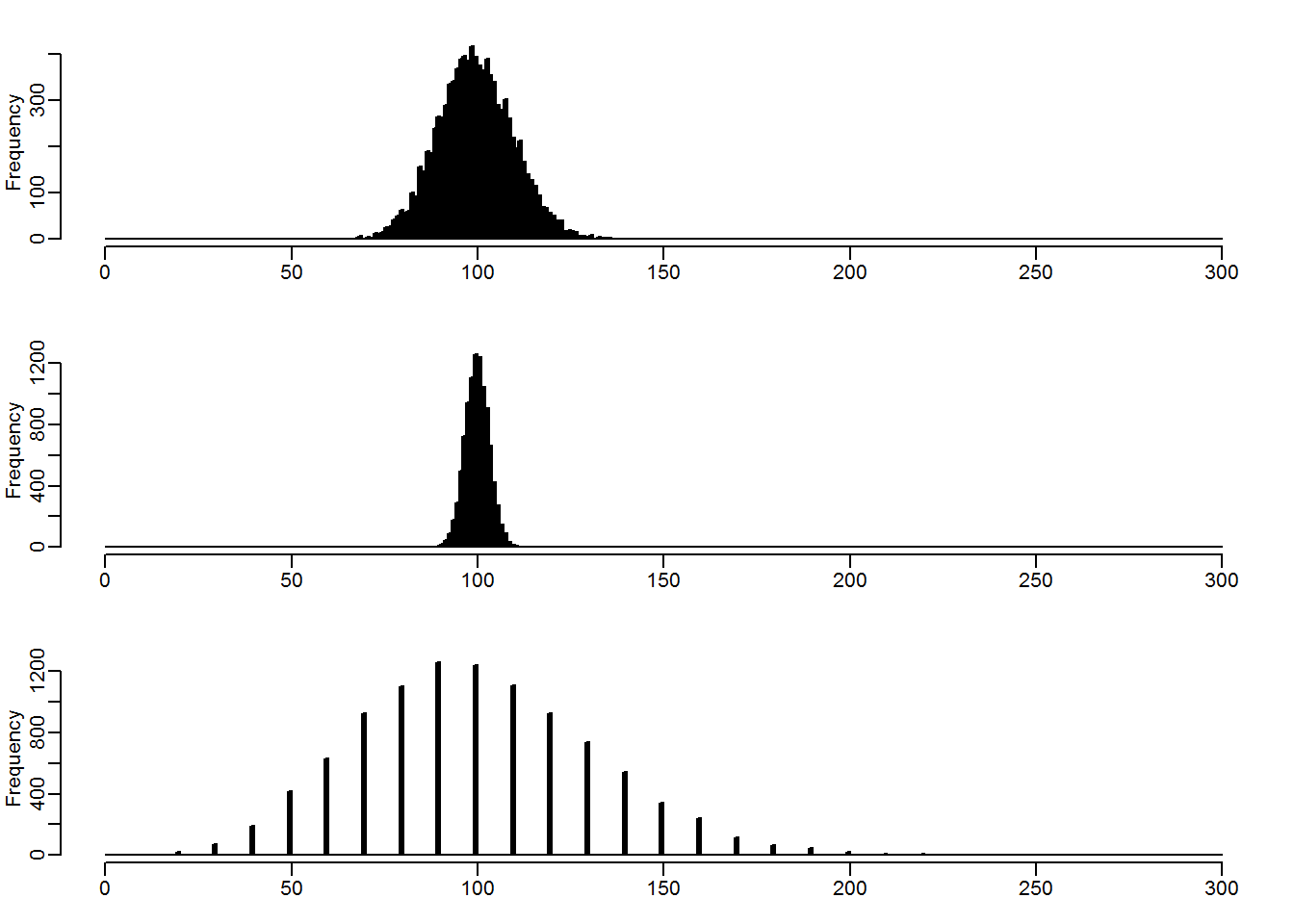data.frame("Base.100" = c(mean(rpois(n,100)), sd(rpois(n,100))),
"1000.to.100" = c(mean(rpois(n,1000)/10), sd(rpois(n,1000)/10)),
"10.to.100" = c(mean(rpois(n,10)*10), sd(rpois(n,10)*10)),
row.names = c("mean", "sd"))
##        Base.100 X1000.to.100 X10.to.100
## mean 100.038300    99.981190   99.76400
## sd     9.943501     3.150362   31.86749
• Once you introduce the possibility of scaled counts/variance, it becomes unclear what the true distribution of a given variable is
• I.e. a gene with a mean of 100 could derive from any of the three plots above (or more), even though only one would be the true distribution

### Differential gene expression with DESeq2

Initializing DESeq2 workflow

library(airway)
library(DESeq2)
data("airway")
dds <- DESeqDataSet(airway, design= ~ cell + dex)

Differential expression output

library(DESeq2)
dds$dex <- relevel(dds$dex, "untrt") #Ensures that "untrt" is the base level for expression
dds <- DESeq(dds)
res <- results(dds)
head(res)
## log2 fold change (MAP): dex trt vs untrt
## Wald test p-value: dex trt vs untrt
## DataFrame with 6 rows and 6 columns
##                    baseMean log2FoldChange      lfcSE       stat
##                   <numeric>      <numeric>  <numeric>  <numeric>
## ENSG00000000003 708.6021697    -0.37415246 0.09884435 -3.7852692
## ENSG00000000005   0.0000000             NA         NA         NA
## ENSG00000000419 520.2979006     0.20206175 0.10974241  1.8412367
## ENSG00000000457 237.1630368     0.03616686 0.13834540  0.2614244
## ENSG00000000460  57.9326331    -0.08445399 0.24990710 -0.3379415
## ENSG00000000938   0.3180984    -0.08413903 0.15133427 -0.5559813
##                    <numeric>   <numeric>
## ENSG00000000003 0.0001535422 0.001289269
## ENSG00000000005           NA          NA
## ENSG00000000419 0.0655868755 0.197066699
## ENSG00000000457 0.7937652378 0.913855995
## ENSG00000000460 0.7354072485 0.884141561
## ENSG00000000938 0.5782236283          NA

MA plots

• MA plots show each gene by the mean of its counts across all sample vs. it’s fold change between conditions

plotMA(res, ylim=c(-4,4))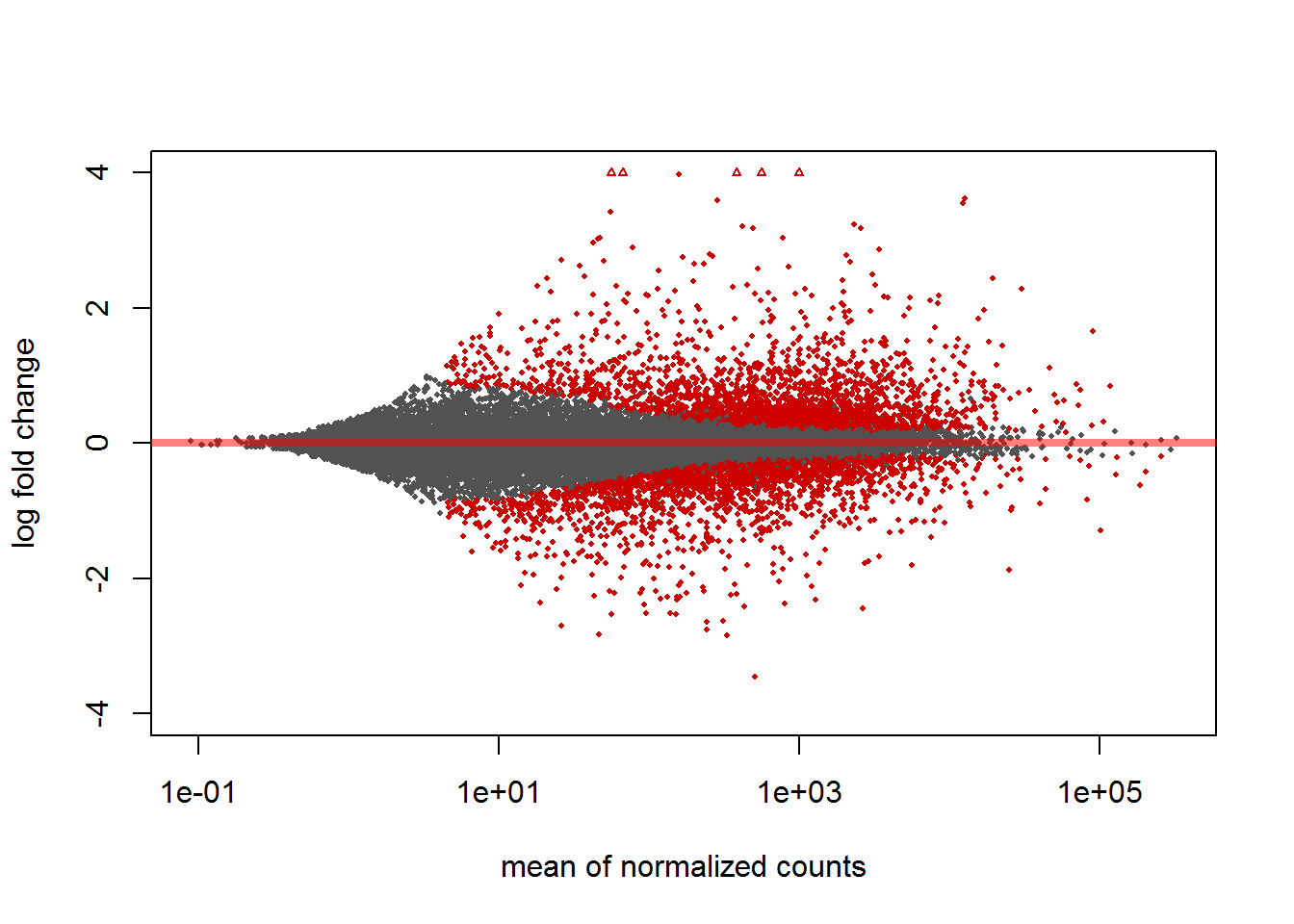• Red dots indicate genes with an adjusted p-value that has acheived significance
• The default alpha is 0.01
• Alpha can be changes in the results function with the argument alpha
library(rafalib)
mypar(1,2)
res2 <- results(dds, alpha=0.05)
plotMA(res, ylim=c(-4,4), main = "alpha=0.1")
plotMA(res2, ylim=c(-4,4), main = "alpha=0.05")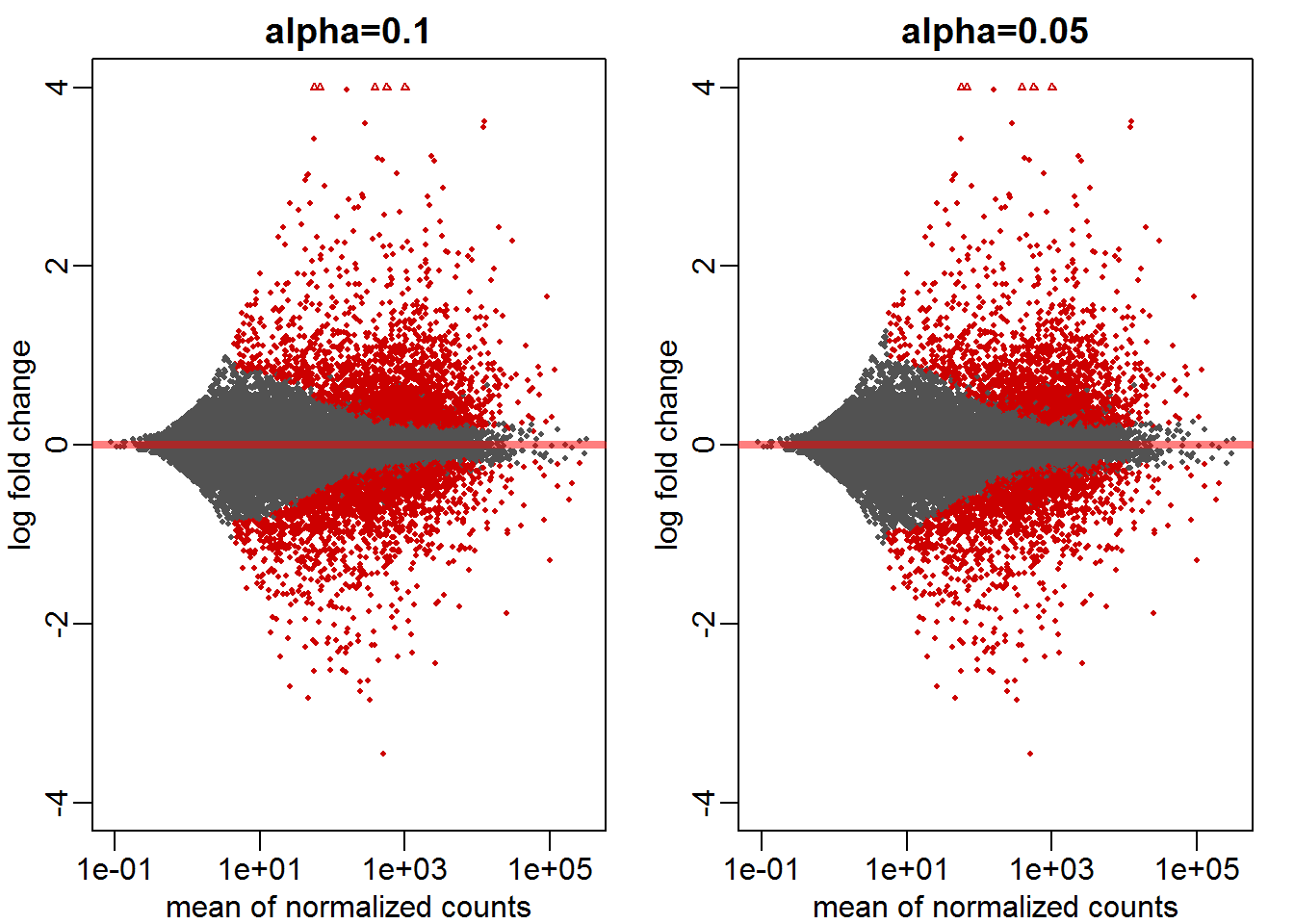• MA plots can also be adjusted to only display significiant differential expression that reaches a fold-change threshold
• Normally, all fold changes which are significant are indicated
• lfcThreshold argument in results can specify a cut off +“lfc” stands for log fold change
library(rafalib)
mypar(1,2)
res3 <- results(dds, lfcThreshold = 1)
plotMA(res, ylim=c(-4,4), main = "no thresh")
plotMA(res3, ylim=c(-4,4), main = "lfcTresh=1")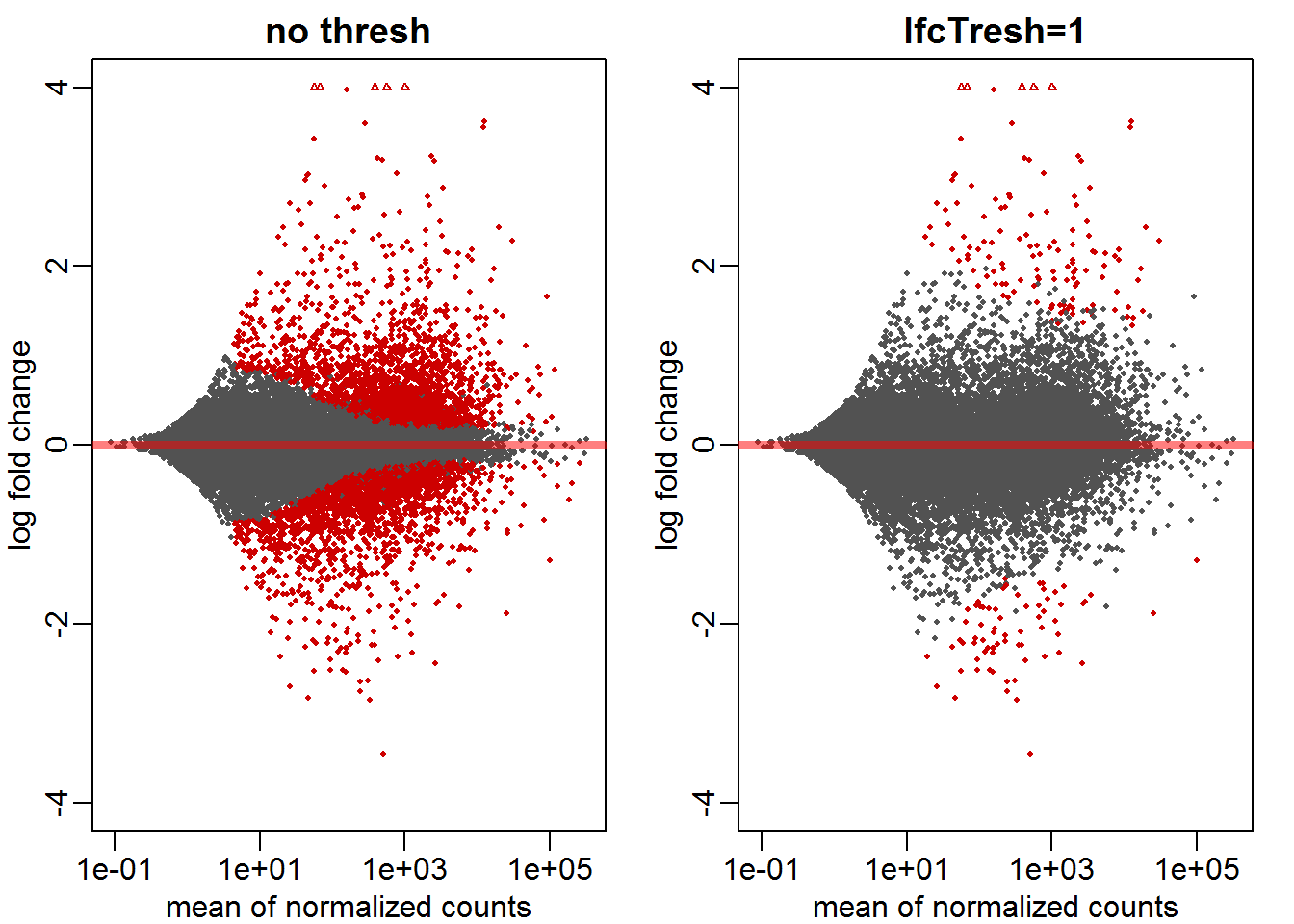Individual genes

*Sorting genes by p-value can highlight important genes

r
resSort <- res[order(res$padj),] head(resSort)   ## log2 fold change (MAP): dex trt vs untrt ## Wald test p-value: dex trt vs untrt ## DataFrame with 6 rows and 6 columns ## baseMean log2FoldChange lfcSE stat ## <numeric> <numeric> <numeric> <numeric> ## ENSG00000152583 997.4398 4.313961 0.1721375 25.06114 ## ENSG00000165995 495.0929 3.186823 0.1281565 24.86665 ## ENSG00000101347 12703.3871 3.618734 0.1489434 24.29604 ## ENSG00000120129 3409.0294 2.871488 0.1182491 24.28337 ## ENSG00000189221 2341.7673 3.230395 0.1366746 23.63567 ## ENSG00000211445 12285.6151 3.553360 0.1579821 22.49216 ## pvalue padj ## <numeric> <numeric> ## ENSG00000152583 1.320082e-138 2.379844e-134 ## ENSG00000165995 1.708459e-136 1.540005e-132 ## ENSG00000101347 2.158519e-130 1.297126e-126 ## ENSG00000120129 2.937761e-130 1.324049e-126 ## ENSG00000189221 1.657244e-123 5.975359e-120 ## ENSG00000211445 4.952520e-112 1.488067e-108  • Can plot expression levels between groups for individual genes library(ggplot2) top.gene <- which.min(res$padj) #selects gene with lowest adjusted pvalue
data <- plotCounts(dds, gene=top.gene, intgroup=c("dex","cell"), returnData=TRUE) #extracts expression data from top.gene
#If returnData=F, would generate a base graphics plot itself
ggplot(data, aes(x=dex, y=count, col=cell, group=cell)) +
geom_point() + geom_line() + scale_y_log10()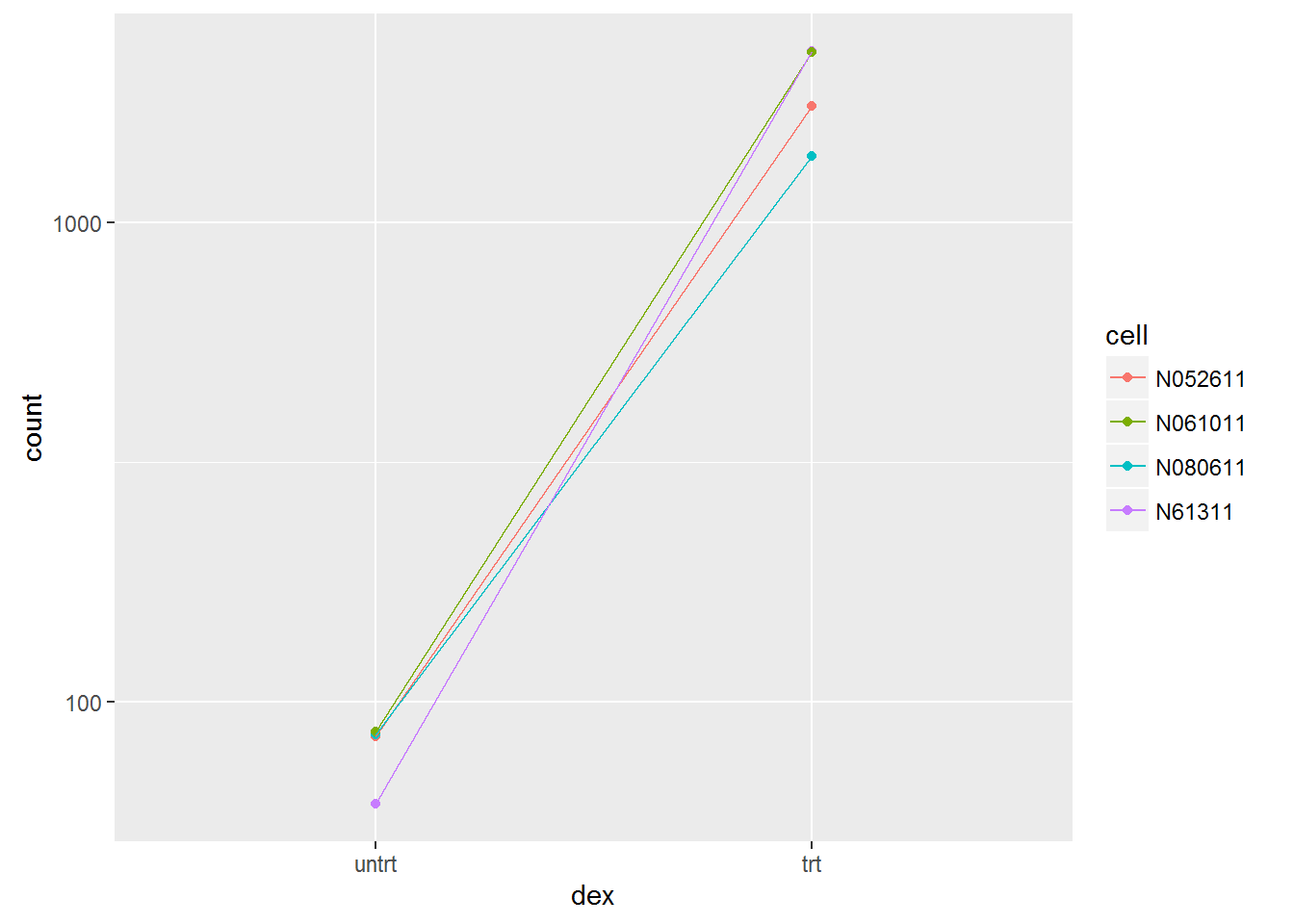Heatmap of top genes

r
# library(pheatmap)